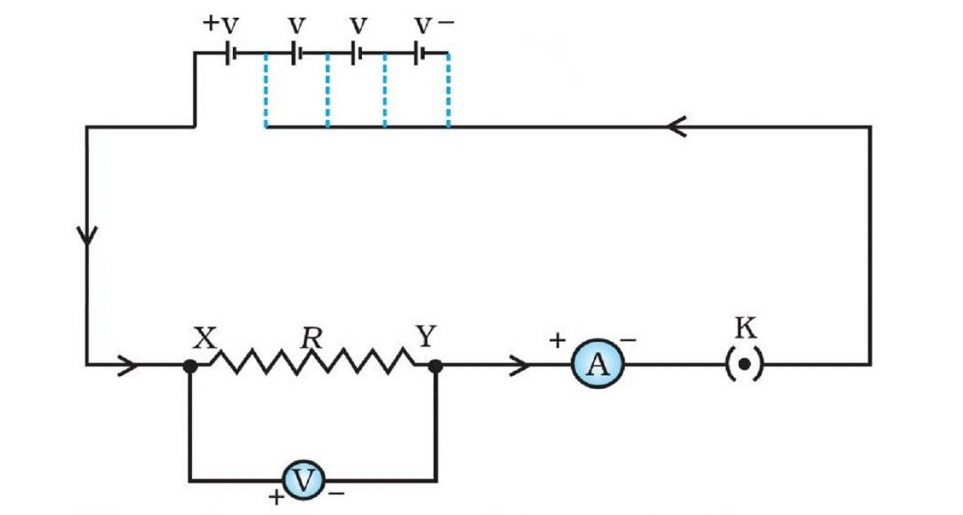# Class 10 Electricity Important Questions : Solutions

Electricity Important Questions : Solutions

1. The potential difference between the terminals of an electric heater is 60 V when it draws a current of 4 A from the source. What current will the heater draw if the potential difference is increased to 120 V.
Sol:
Here, V = 60 V and I = 4 A
Using, R = V/I = 60/4 = 15 Ω
Now, V = 120 V
So, I = V/R = 120/15 = 8 A
2. The V-I graph of three resistors is shown below. Which resistor has maximum resistance?Sol:
Resistor R3 has maximum resistance.
3. An electric iron is marked 400 W and 220 V. What is the resistance when iron is hot? How long could it be used for ₹3 of electrical energy that costs 15 paise per unit.
Sol:
Given, P = 400 W and V = 220 V
Using, P = /R
⇒ R = /P = 220²/400 = 121 Ω
So, resistance when iron is hot = 121 Ω

Now, rate of electrical energy = 15 paise per unit
and total money = ₹3 = 300 paise
So, electrical energy consumed, E = 300/15 = 20 unit = 20 kWh = 20000 Wh
Using, E = P × t
⇒ t = E/P = 20000/400 = 50 h
So, It can be used for 50 hours.
4. A 20 cm long uniform wire of resistance 5 Ω is stretched to a uniform wire of 40 cm length. What will be the resistance of new wire?
Sol:
length = l = 20 cm
Area of cross-section = A
Resistance = R = ρl/A = 5 Ω
New length = l’ = 40 cm = 2l
New area of cross-section = A’
When the wire is stretched, its volume remains the same.
So, A’ × l’ = A × l
⇒ A’ × 40 = A × 20
⇒ A’ = A/2
New resistance
= R’
ρl’/A’
ρ(2l)/(A/2)
= 4ρl/A
= 4R = 4 × 5 = 20 Ω
Therefore, resistance of the new wire is 20 Ω.
5. If R1 and R2 are the resistances of filaments of a 400 W and a 200 W lamp, designed to operate on the same voltage, then find the relationship between R1 and R2.
Sol:
For R1, P1 = 400 W
For R2, P2 = 200 W
Using, P = /R
(V1)2 = P1 x R1 = 400R1
and (V2)2 = P2 x R2 = 200R2

Since, both filaments operate on the same voltage.
So, (V1)2 = (V2)2
⇒ 400R1 = 200R2
⇒ 2R1 = R2
6. .
1. State Ohm’s law and write its expression.
Sol:
According to Ohm’s law, at constant temperature, the potential difference across the ends of a conductor is directly proportional to the amount of current flowing through it.
Mathematically,
V ∝ I
V = IR where, R is constant of proportionality & is known as resistance.
2. Draw a circuit diagram to verify Ohm’s law.
Sol: Circuit diagram for the verification of Ohm’s law:7. The rating of bulb B1 is 60 V, 12 W & bulb B2 is 100 V, 100 W. Find the maximum emf of the battery so that all bulbs remain safe.Sol:
For B1,
V1 = 60 V, P1 = 120 W
R1V1²/P1 = 60²/120 = 300 Ω
For B2,
V2 = 100 V, P2 = 100 W
R2V2²/P2 = 100²/100 = 100 Ω
Since, B1 and B2 are connected in parallel.
So, 1/Rp = 1/R1 + 1/R2 = 1/300 + 1/100 = 4/300
⇒ Rp = 300/4 = 75 Ω
So, Req across circuit = 75 + 300 + 25 = 400 Ω

Current that can flow through B1 = V1/R1 = 60/300 = 1/5 A
Current that can flow through B2 = V2/R2 = 100/100 = 1 A
So, maximum current that can flow through the circuit so that all bulbs remain safe = 1/5 A

Therefore, required e.m.f. = IReq = 1/5 x 400 = 80 V
8. Find equivalent resistance between P and Q:Sol:9. Find equivalent resistance between P and Q:Sol:
Here, all resistors are connected in parallel.
So, 1/Rp = 1/R1 + 1/R2 + 1/R3 = 1/10 + 1/10 + 1/10 = 3/10
⇒ Rp = 10/3 Ω
10. In reference to the given figure, find the current flowing through 20 Ω resistor.Sol:
RAB = R1 + R2 = 5 + 20 = 25 Ω
RCD = R1 + R2 = 10 + 40 = 50 Ω

1/Req = 1/RAB + 1/RCD = 1/25 + 1/50 = 3/50
⇒ Req = 50/3 Ω

I = 3 A
So, V = IReq = 3 x 50/3 =50 V

In parallel, voltage remains same.
So, current flowing through AB, I = V/RAB = 50/25 = 2 A

In series, current flowing through each resistor remains same.
So, current flowing through 20 Ω resistor = 2 A.

• ##### Shivansh preet

If u want to being motivated in your life,

3
2